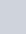A vector vector r is inclined at equal angles to the three axes.
Question:

A vector $\vec{r}$ is inclined at equal angles to the three axes. If the magnitude of $\vec{r}$ is $2 \sqrt{3}$ units, find $\vec{r}$

Solution:

As the vectormakes equal angles with the axes, their direction cosines should also be same

So, l = m = n

And we know that,

l2 + m2 + n= 1 ⇒ l2 + l2 + l2 = 1

3l2 = 1

l = ± 1/√3